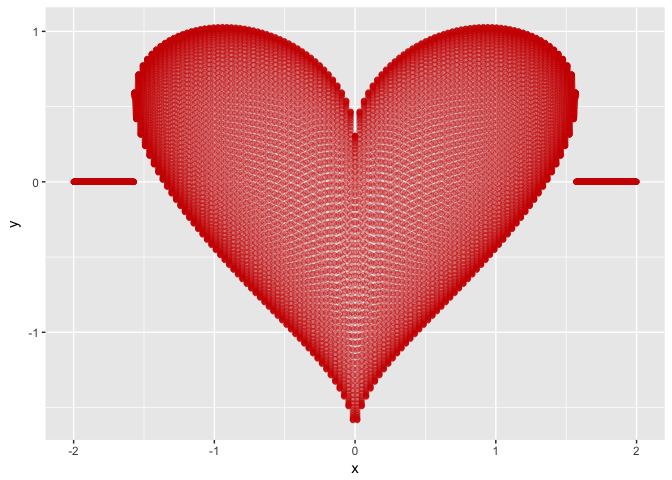Wed 15 November 2017 | tags: Scientific Computing, R, -- (permalink)

I have recently helped a PhD student read and merge about 150 CSV files. I used R, but the student wanted to use Stata later, so I used the haven package to export to the Stata 14 native file format.

There is nothing to report about the process everything was quick and easy and worked as expected. But I noticed that .dta file (Stata file) was substantially larger than the original data. The original data CSV files were a little above 8GB, the consolidated R file was about 1.3GB (no surprises here as R saves the files in a ...

Mon 25 September 2017 | tags: Scientific Computing, R, -- (permalink)

Scientific computation is nowadays an integral part of most research in many science fields. But the majority of researchers never had any formal training on how to structure, maintain, and collaborate on such projects. I had to learn the hard way, and there are several resources nowadays that I wished I had access to when I was starting.

A good start is to read Good enough practices in scientific computing paper, and Best Practices for Scientific Computing. They provide apt recommendations from data management, collaboration, project organisation, revision control systems to the writing of manuscripts.

I also recommend The Plain ...

Mon 11 September 2017 | tags: R, -- (permalink)

A couple of days ago I was giving a course on R, and I used the following example\footnote{This function calculates the value of an American call option using a binomial tree.}:

```library(fOptions)
system.time(CRRBinomialTreeOption(TypeFlag = "ca", S = 50, X = 50, Time = 5/12, r = 0.1, b = 0.1, sigma = 0.4, n = 2000)@price)
```
```##    user  system elapsed
##   7.807   0.034   8.002
```

I was puzzled that on my laptop it took about 8 seconds to compute and on the lab computers it took less the 2 seconds. Since my laptop has a relatively recent ...

Sat 03 June 2017 | tags: R, -- (permalink)

Yesterday a colleague told me that he was surprised by the speed differences of R, Julia and Stata when estimating a simple linear model for a large number of observations (10 million). Julia and Stata had very similar results, although Stata was faster. But both Julia and Stata were substantially faster than R. He is an expert and long time Stata user and was sceptical about the performance difference. I was also surprised. My experience is that R is usually fast, for interpreted language, and most procedures that are computationally intensive are developed in a compiled language like C, Fortran ...

Fri 26 May 2017 | tags: R, -- (permalink)

Recently Mike Croucher blogged about Microsoft Azure’s free Jupyter notebooks. He showed that the computational power provided by Microsoft Azure Notebooks is quite considerable. This is a free cloud service, and Jupyter notebooks power the interface. We can use within those notebooks both Python (2.7 and 3.5.1), F#, and R (3.3), and we have the ability to install packages if needed. 2

Although there is a 4Gb memory limit, the notebook has access to fast processors, eight in fact.1 I was curious to see if the service allowed parallel computing, and to my surprise ...

Sat 29 April 2017 | tags: R, -- (permalink)

In the previous post I have described the first five functions, introduced by dplyr 0.5, that are listed below:

• coalesce()
• case_when()
• if_else()
• na_if()
• near()
• recode()
• union_all()
• summarise_all() and mutate_all()
• summarise_at() and mutate_at()
• summarise_if() and mutate_if()
• select_if()

In this post, I’ll describe the others. Meanwhile, the next version of dplyr is just around the corner, and will also bring new features.

## `recode()`

The `recode()` function, as the name states, allow the recoding of a vector of values. There is also a similar function for factors: `recode_factor()`.

Let’s take the following `data_frame`:

```library(dplyr)
d_f <- data_frame(x = c(1 ...```

Sat 11 March 2017 | tags: R, -- (permalink)

dplyr version 0.5 introduced several new functions:

• coalesce()
• case_when()
• if_else()
• na_if()
• near()
• recode()
• union_all()
• summarise_all(), mutate_all()
• summarise_at() and mutate_at()
• summarise_if() and mutate_if()
• select_if()

Let’s take a look at the first five.

## `coalesce()`

```library(dplyr)
```

The `coalesce()` function takes two or more vectors as arguments and finds the first non-missing value at each position. It serves a similar purpose as the COALESCE SQL function.

It is easy to illustrate what the function does with a simple example:

```y <- c(NA, 2, NA, NA, 5)
z <- c(NA, NA, 3, 4, NA)
w <- c(10, 20, 30, NA, NA)
coalesce ...```

Tue 14 February 2017 | tags: R, -- (permalink)

There are several ways of plotting a heart shaped function. The following is a simple one using ggplot2:

```library(ggplot2)

heart <- function(x) {
h <- suppressWarnings(sqrt(cos(x))*cos(200*x) + sqrt(abs(x))
- 0.7*(4 - x^2)^0.01)
h[which(is.nan(h))] <- 0
return(h)
}

ggplot(aes(x), data = data.frame(x = c(-2,2))) +
stat_function(fun = heart, color="red3",
geom = "point", n = 15000, alpha=0.3)
```Fri 20 January 2017 | tags: R, -- (permalink)

Hadley Wickham’s universe of packages along with pipes (%>%) from the magrittr package has transformed the way I use R. They create a new dialect for R and provide a large set of tools for data manipulation and visualisation.

They were formerly and informally known as Hadleyverse, but the author prefers the term tidyverse. This set of packages can now be installed and loaded using a wrapper package called tidyverse.